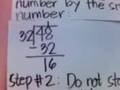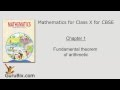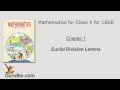Euclid’s Division Lemma  ( 1)

Questions

Q. Problem no. 4 in exercise ... 5, 001 answer under Euclid’s Division Lemma 9 years, 3 months ago

A. any positive integer n can be written as 3q + r where q is the quotient and r the remainder using euclid's division algo n=aq+r (here a=3) so n = 3q + r q is in a integer and r = 0/1/2 n^2 =... link
9 years, 3 months ago
answer

Q.
``````what is euclid's division lemma?
``````
2, 001 answer under Euclid’s Division Lemma 8 years, 7 months ago

A. for 2 no a and b there exists integers b and q such that a=bq+r. a,b = integers link
8 years, 7 months ago
answer

Q. Euclid's division lemma is quite attractive and new to me. Please continue to share such useful information. fnaf world ... 1, 000 answers under Euclid’s Division Lemma 6 months, 1 week ago
answer

Q. Euclid's division lemma is quite attractive and new to me. Please continue to share such useful information. fnaf world ... 1, 000 answers under Euclid’s Division Lemma 6 months, 1 week ago
answer

Videos